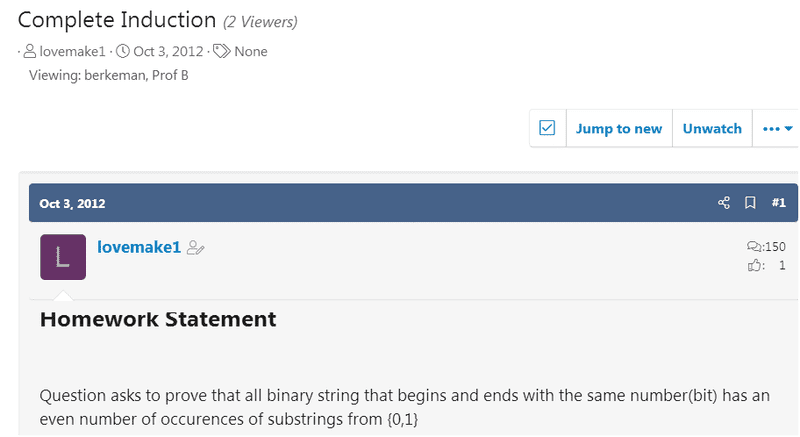# Complete Induction -- How to remove my subscription to this very old thread?

lovemake1

## Homework Statement

Question asks to prove that all binary string that begins and ends with the same number(bit) has an even number of occurences of substrings from {0,1}

Hint: you may find useful to combine claims about strings that start and end with different bit

## Homework Equations

{0} = {} since 0 is a multiple of 2 it holds.
{11} = {} since 0 is a multiple of 2 it holds.
eg 010010 = (01), (10), (01) (10)

## The Attempt at a Solution

My induction hypothesis was, for all natural number n, assume that p(j) holds for some integer j such that 0 <= j < n, that is a binary string with length j that begins and ends with the same bit has an even number of ooccurences of substrings from {0,1}

so, let j = n - 1 and by I.H we know that p(j) holds.
j, begins and ends with the same bit. and can be represented like so, P(j) = 2t for some integer multiple t. since 1st and last digit of j is the same, we know if n = jlast then there are no additional sets created so the equation will still be p(n) = 2t + 0;

but if we were to add n != jlast, then there would be 1 extra subset at the end since ...01 or ...10 will take place. therefore, the equation will now be p(n) = 2t + 1; even number + odd = odd. hence we've shown that for all n, if first and last digit of string is the same, there are even number of substrings from {0,1}

I feel like I have done too much explaining in english and not enough mathenatical proof.
is there somewhere I went too far? help is appreciated.

Last edited:
•Delta2

Prof B
@moderators Short of ignoring the OP, is there any way to make this (formerly) unanswered question disappear from my feed? The question makes no sense. The homework equations make no sense. The attempted answer makes no sense. The OP has not been seen in 9 years.

Mentor
@moderators Short of ignoring the OP, is there any way to make this (formerly) unanswered question disappear from my feed? The question makes no sense. The homework equations make no sense. The attempted answer makes no sense. The OP has not been seen in 9 years.

You should be able to just click the Unwatch link in the upper right corner of the thread. Does that work for you?Prof B
I see now. Thank you.

•berkeman